# Law of the Lever: Definition, Formula & Examples

Lesson Transcript
Instructor: Matthew Bergstresser

Matthew has a Master of Arts degree in Physics Education. He has taught high school chemistry and physics for 14 years.

One of the six simple machines is the lever. Levers allow a relatively smaller force to move a heavier object. In this lesson, we will investigate the law of the lever and work some examples involving levers.

## Uses of the Lever

Have you ever been on a seesaw or opened a paint can's lid? If so, you were using a lever, which is a bar or other rigid object that has a point to pivot around. The pivot point is called a fulcrum.

Let's say you're on a seesaw with your friend who weighs a lot more than you and you want the seesaw to be balanced. You could position yourself at a certain distance from the fulcrum so your lighter bodyweight counters your friend's bodyweight, allowing the seesaw to be in equilibrium. This means there's no rotation. Let's see how the math works when dealing with a lever.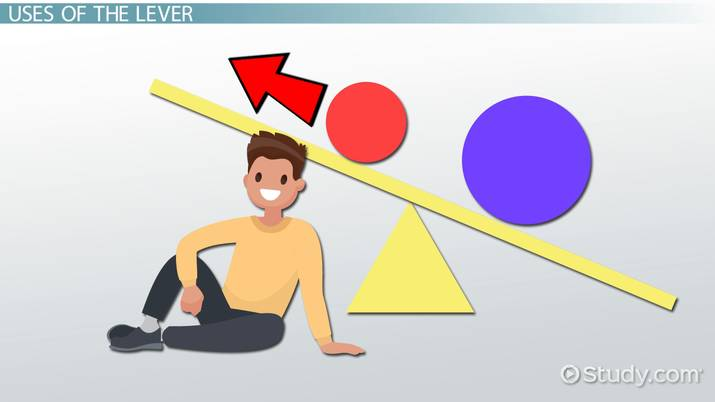An error occurred trying to load this video.

Try refreshing the page, or contact customer support.

Coming up next: Critical Thinking and Logic in Mathematics

### You're on a roll. Keep up the good work!

Replay
Your next lesson will play in 10 seconds
• 0:04 Uses of the Lever
• 0:37 The Law of the Lever
• 2:25 Example 1
• 3:30 Example 2
• 4:43 Lesson Summary
Save Save

Want to watch this again later?

Timeline
Autoplay
Autoplay
Speed Speed

## The Law of the Lever

The cross product of force and distance is torque. The cross product is the mathematical process between two vectors that results in a vector perpendicular to both of the initial vectors. The law of the lever is also known as the law of moments and equates clockwise torques and counterclockwise torques. The equation here shows the law of levers: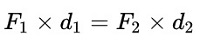• F1 is force 1.
• d1 is the distance from the fulcrum force 1 is applied.
• F2 is force 2.
• d2 is the distance from the fulcrum force 2 is applied.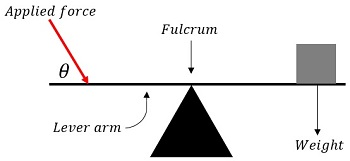Imagine trying to open a paint can's lid with a screwdriver, but not applying the force perpendicularly to the screwdriver. If you applied the force at 1° to the screwdriver, it would take a lot of force to open the lid. Applying the force at 90° to the screwdriver would be the most effective at prying open the lid.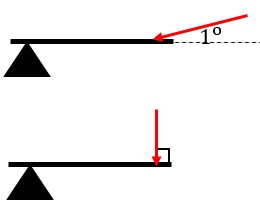This means we need a way to adjust the amount of torque based on the angle at which the force is applied to the lever. The sine trigonometric function has a maximum value at 90° and a minimum value at 0°. We can rewrite the cross product of force and distance from the lever arm including the sine function. This is how the cross product is evaluated. The equation for the magnitude of torques in the law of levers: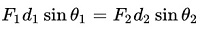• θ1 is the angle between F1 and the lever arm.
• θ2 is the angle between F2 and the lever arm.

We'll deal with situations where the angle between the force and the lever arm is 90° so we can remove the sine functions because the sin of 90° is 1. This gives us this next equation: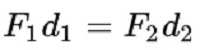Now, we can work some practice problems using the law of levers. Let's get to it!

## Example 1

Imagine Sal weighs 150 pounds, and his friend Joe weighs 100 pounds. Sal sits 3 feet from the fulcrum on a seesaw. How far does Joe need to sit on the other side of the fulcrum so the seesaw is in equilibrium?

The first step in solving this problem is to draw a diagram, which we'll call Diagram 1.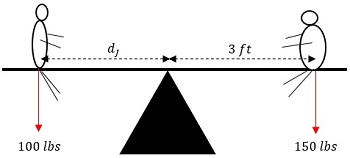Now we can use this next equation to determine how far Joe needs to sit from the fulcrum so there's no rotation of the board they're sitting on.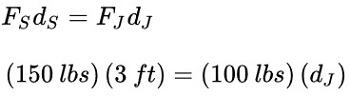• FS is the weight of Sal.
• FJ is the weight of Joe.
• dS is the distance Sal is from the fulcrum.
• dJ is how far Joe has to be from the fulcrum to put the seesaw in equilibrium.

When we plug in our values, we get 150lbs x 3ft = 100lbs x dJ.

Now we can divide both sides by 100lbs to get the distance Joe needs to sit from the fulcrum to put the seesaw in equilibrium. We'll call this Step 3.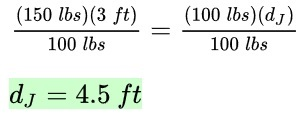We see that our answer, our dJ, equals 4.5ft.

To unlock this lesson you must be a Study.com Member.

### Register to view this lesson

Are you a student or a teacher?